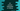# C# program to convert Fahrenheit to Celsius## C# program to convert Fahrenheit to Celsius:

In this program, we will learn how to convert a Fahrenheit value to Celsius in C#. We will take the fahrenheit value as an input from the user and convert it to celsius.

With this program, you will learn how to read user inputs and how to do simple mathematical calculations in C#.

### Formula to convert fahrenheit to celsius:

Below formula is used to convert a Fahrenheit value to Celsius:

``Celsius = (Fahrenheit - 32) * (5/9)``

### C# program:

Let’s write the C# program:

``````using System;

public class Program
{
public static void Main()
{
double fahrenheit, celsius;

Console.WriteLine("Enter the Fahrenheit value : ");

celsius = (fahrenheit - 32) * (5/9);

Console.WriteLine("Celsius : {0}",celsius);
}
}``````

Here,

• fahrenheit and celsius are double variables to store the Fahrenheit and Celsius values.
• We are taking the fahrenheit as user input and storing it in the variable.
• celsius is calculated based on the fahrenheit value.

It will print results as like below:

``````Enter the Fahrenheit value :
32
Celsius : 0``````